Difference between revisions of "Lennard-Jones model"

The Lennard-Jones intermolecular pair potential is a special case of the Mie potential and takes its name from Sir John Edward Lennard-Jones (Ref. 1). The Lennard-Jones model consists of two 'parts'; a steep repulsive term, and smoother attractive term, representing the London dispersion forces. Apart from being an important model in its-self, the Lennard-Jones potential frequently forms one of 'building blocks' of may force fields,

Functional form

The Lennard-Jones potential is given by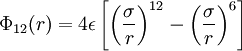$\Phi_{12}(r) = 4 \epsilon \left[ \left(\frac{\sigma}{r} \right)^{12}- \left( \frac{\sigma}{r}\right)^6 \right]$

where

•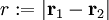$r := |\mathbf{r}_1 - \mathbf{r}_2|$
•$\Phi_{12}(r)$ is the intermolecular pair potential between two particles or sites
•$\sigma$ is the diameter (length), i.e. the value of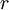$r$ at which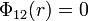$\Phi_{12}(r)=0$
•$\epsilon$ is the well depth (energy)

In reduced units:

• Density: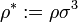$\rho^* := \rho \sigma^3$, where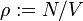$\rho := N/V$ (number of particles$N$ divided by the volume$V$)
• Temperature: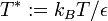$T^* := k_B T/\epsilon$, where$T$ is the absolute temperature and$k_B$ is the Boltzmann constant

The following is a plot of the Lennard-Jones model for the parameters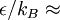$\epsilon/k_B \approx$ 120 K and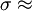$\sigma \approx$ 0.34 nm. See argon for different parameter sets.

This figure was produced using gnuplot with the command:

plot (4*120*((0.34/x)**12-(0.34/x)**6))

Special points

•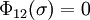$\Phi_{12}(\sigma) = 0$
• Minimum value of$\Phi_{12}(r)$ at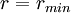$r = r_{min}$;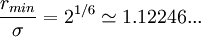$\frac{r_{min}}{\sigma} = 2^{1/6} \simeq 1.12246 ...$

Critical point

The location of the critical point is (Caillol (Ref. 2))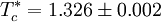$T_c^* = 1.326 \pm 0.002$

at a reduced density of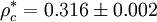$\rho_c^* = 0.316 \pm 0.002$.

Vliegenthart and Lekkerkerker (Ref. 4) have suggested that the critical point is related to the second virial coefficient via the expression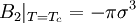$B_2 \vert_{T=T_c}= -\pi \sigma^3$

Triple point

The location of the triple point as found by Mastny and de Pablo (Ref. 3) is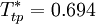$T_{tp}^* = 0.694$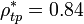$\rho_{tp}^* = 0.84$ (liquid);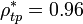$\rho_{tp}^* = 0.96$ (solid)

Approximations in simulation: truncation and shifting

The Lennard-Jones model is often used with a cutoff radius of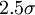$2.5 \sigma$. See Mastny and de Pablo (Ref. 3) for an analysis of the effect of this cutoff on the melting line.

m-n Lennard-Jones potential

It is relatively common to encounter potential functions given by: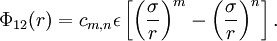$\Phi_{12}(r) = c_{m,n} \epsilon \left[ \left( \frac{ \sigma }{r } \right)^m - \left( \frac{\sigma}{r} \right)^n \right].$

with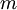$m$ and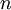$n$ being positive integers and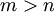$m > n$.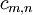$c_{m,n}$ is chosen such that the minimum value of$\Phi_{12}(r)$ being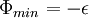$\Phi_{min} = - \epsilon$. Such forms are usually referred to as m-n Lennard-Jones Potential. For example, the 9-3 Lennard-Jones interaction potential is often used to model the interaction between the atoms/molecules of a fluid and a continuous solid wall. On the '9-3 Lennard-Jones potential' page a justification of this use is presented.

The following plot is of a typical radial distribution function for the monatomic Lennard-Jones liquid (here with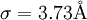$\sigma=3.73 {\mathrm {\AA}}$ and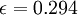$\epsilon=0.294$ kcal/mol at a temperature of 111.06K:

1. John G. Kirkwood, Victor A. Lewinson, and Berni J. Alder "Radial Distribution Functions and the Equation of State of Fluids Composed of Molecules Interacting According to the Lennard-Jones Potential", Journal of Chemical Physics 20 pp. 929- (1952)

Equation of state

Main article: Lennard-Jones equation of state

Virial coefficients

Main article: Lennard-Jones model: virial coefficients

Phase diagram

Main article: Phase diagram of the Lennard-Jones model